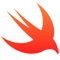/ Database

# Swift library to make easy using local document-oriented database in iOS apps## StorageDone-iOS

Swift library to make easy use local document-oriented databases in iOS apps.

### Installation

``````pod 'StorageDone'
``````

### Usage

StorageDone lets you save Codable models in a local database very easily.

First create a model

``````struct Teacher: Codable {
let id: String
let name: String?
let surname: String?
let age: Int?
let cv: String?
}
``````

Then create a `StorageDoneDatabase` object and save an instance of a Codable model in it

``````let teacher = Teacher(id: "id1", name: "Sarah", surname: "Jones", age: 29, cv: "https://my.cv.com/sarah_jones")
let database = StorageDoneDatabase(name: "teachers")

try? database.insert(element: teacher)
``````

Reading database content will retrieve an array of the decleared model

``````do {
let savedTeachers: [Teacher] = try database.get()
} catch let e {
print(e)
}
``````

Other methods allow filtering and deletion.

### Primary key

A model can implement `PrimaryKey` protocol, in order to have an attribute set as database primary key

``````struct Teacher: Codable, PrimaryKey {
let id: String
let name: String?
let surname: String?
let age: Int?
let cv: String?

func primaryKey() -> String {
return "id"
}
}
``````

Primary keys come in combination with insert or update methods

``````let teachers = [Teacher(id: "id1", name: "Sarah", surname: "Jones", age: 29, cv: "https://my.cv.com/sarah_jones"),
Teacher(id: "id2", name: "Silvia", surname: "Jackson", age: 29, cv: "https://my.cv.com/silvia_jackson"),
Teacher(id: "id3", name: "John", surname: "Jacobs", age: 30, cv: "https://my.cv.com/john_jackobs")]

try? database.insertOrUpdate(elements: teachers)
``````

### RxSwift

Every operation has its RxSwift version. Each can be used through rx extension

``````
database.rx.insertOrUpdate(teachers)

database.rx.insert(teachers)

database.rx.get()

database.rx.get(["id":"id1"])

database.rx.delete(["id":"id2"])

database.rx.deleteAllAndInsert(teachers)

``````

### Operators

Database objects can use different custom operators, which wrap try-catch logic and give a more compact way to access database

``````// Insert or update
database ++= teachers

let teachers: [Teacher] = <-database

// Filter
let filteredTeachers: [Teacher] = ["id":"id1"] <- database

// Delete if model implements PrimaryKey protocol
database --= teachers
``````

### Queries

Get and delete commands can use queries. Queries can be built in different ways, using custom operator or extensions on parameter name

``````
// Equal
"id" *== "id1"
"id".equal("id1")

// Comparison (Numeric only)
"age" *> 20
"age".greaterThan(20)

"age" *>= 20
"age".greaterThanOrEqual(20)

"age" *< 20
"age".lessThan(20)

"age" *<= 20
"age".lessThanOrEqual(20)

"age" <=&&<= (10, 20)
"age".between((10, 20))

// Is nil
*?"name"
"name".isNil

// Is not nil
*!"name"
"name".isNotNil

// Value inside array
"id" |> ["id1", "id2", "id3"]
"id".inside(["id1", "id2", "id3"])

// Array contains value
"array" |< "A1"
"array".contains("A1")

// Like
"name" **= "A%"
"name".like("A%")

// Regex
"city" /== "\\bEng.*e\\b"
"city".regex("\\bEng.*e\\b")

// Dates comparisons
"dateCreated" *> Date()
"dateCreated".greaterThan(Date())

"dateCreated" *>= Date()
"dateCreated".greaterThanOrEqual(Date())

"dateCreated" *< Date()
"dateCreated".lessThan(Date())

"dateCreated" *<= Date()
"dateCreated".lessThanOrEqual(Date())

// And
and(expression1, expression2, expression3)

// Or
or(expression1, expression2, expression3)

// Usage
do {
let teachers: [Teacher] = try database.get(expression)
} catch let e {
print(e)
}
``````

## Live queries

Using live queries it's possible to observe database changes.

``````// All elements
let liveQuery = try storage.live(Teacher.self) {
teachers in
print("Count \(teachers.count)")
}

let liveQuery = try storage.live {
(teachers: [Teacher]) in
print("Count \(teachers.count)")
}

// Elements with query
let liveQuery = try storage.live(Teacher.self, expression: "id".equal("id1")) {
teachers in
print(teachers)
}

let liveQuery = try storage.live("id".equal("id1")) {
(teachers: [Teacher]) in
print(teachers)
}
``````

In order to stop observing just call cancel on LiveQuery object.

``````liveQuery.cancel()
``````

### RxSwift live queries

Live queries are also available through RxSwift extensions.

``````// All elements
let disposable = database.rx.live(Teacher.self).subscribe(onNext: {
teachers in
print("Count \(teachers.count)")
})

let disposable = database.rx.live().subscribe(onNext: {
(teachers: [Teacher]) in
print("Count \(teachers.count)")
})

// Elements with query
let disposable = database.rx.live(Teacher.self, expression: "id".equal("id1")).subscribe(onNext: {
teachers in
print("Count \(teachers.count)")
})

let disposable = database.rx.live("id".equal("id1")).subscribe(onNext: {
(teachers: [Teacher]) in
print("Count \(teachers.count)")
})
``````

To stop observing changes just dispose the disposable or alternatively add it to a dispose bag.

``````disposable.dispose()

// or

disposable.disposed(by: disposeBag)
``````

Using advanced queries lets to specify filtering expression, ordering logic and priority, limit and skip values.
All of these parameters are optional. The only limitation is that skip is ignored if limit parameter is not present.

``````
try database.get {
\$0.expression = or("id".equal("id1"), "name".equal("Silvia"), "name".equal("John"))
\$0.orderings = ["name".ascending, "date".descending]
\$0.limit = 3
\$0.skip = 2
}

let teachers: [Teacher] = {
\$0.expression = or("id".equal("id1"), "name".equal("Silvia"), "name".equal("John"))
\$0.orderings = ["name".ascending, "date".descending]
\$0.limit = 3
\$0.skip = 2
} <- databaseCore

try database.live({
\$0.expression = or("id".equal("id1"), "name".equal("Silvia"), "name".equal("John"))
\$0.orderings = ["name".ascending, "date".descending]
\$0.limit = 3
\$0.skip = 2
}) {
(liveTeachers: [Teacher]) in
print("Count \(liveTeachers.count)")
}
``````

## Author

Dario Pellegrini, [email protected]

## GitHub#### iOS Example

A nice collection of often useful examples done in Objective-C and Swift.

Latest
Tag